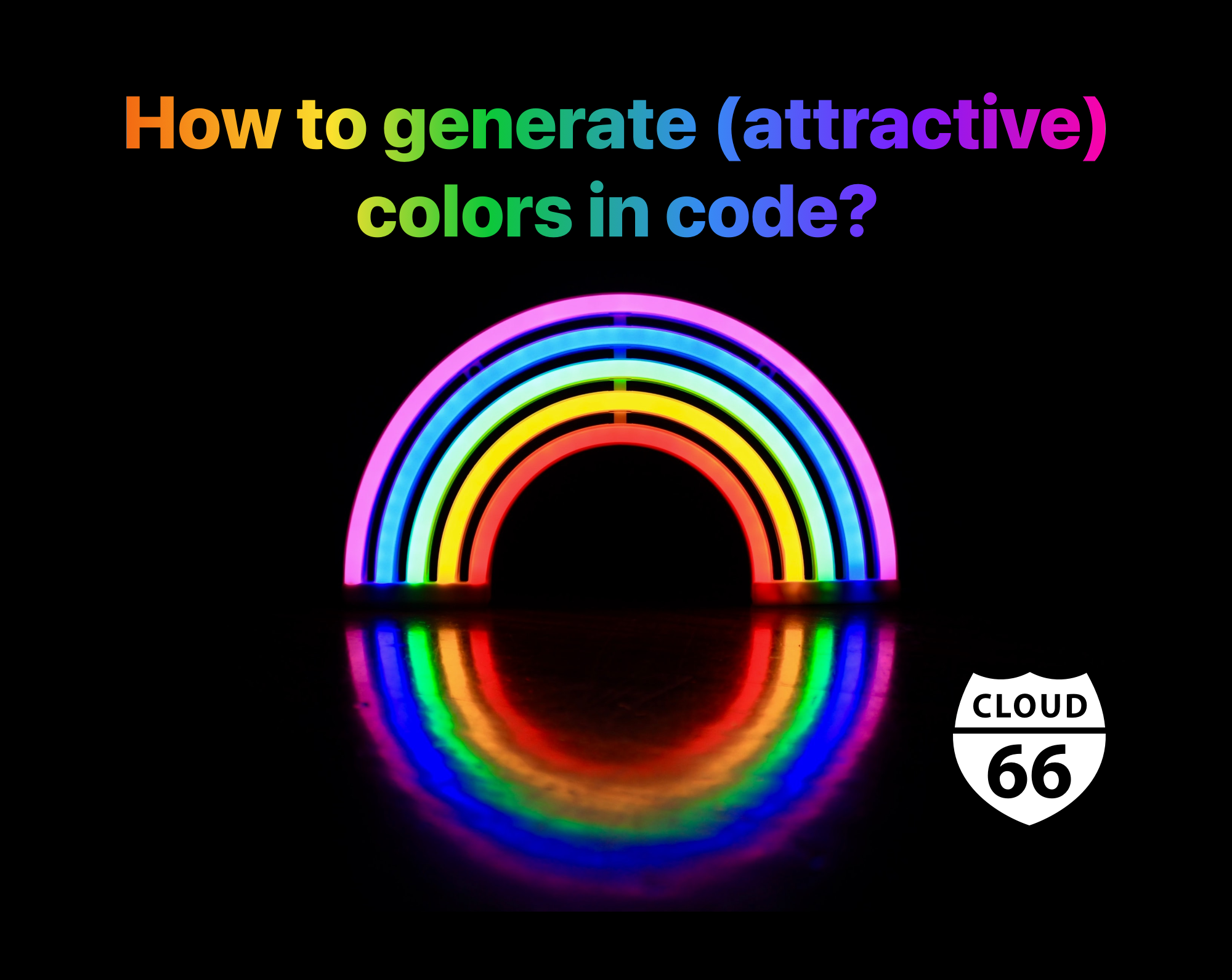← All Articles

# How to generate (attractive) colors in code?Khash SajadiNot all colors look good. Let me rephrase: not all colors look good on everything. This is even more applicable when it comes to websites. When put next to each other, it is important for colors to look good, have the right contrast and be readable. Thankfully there are ways to generate such colors - attractive, readable and complementary - using code.

I started looking into generating attractive and complementary colors when we were working on a feature involving tags. We wanted to let users choose the color of their tags, and not only help them choose a good looking color, but also automatically derive variations of their color for the best visual effect. Take a look at this:The text and the border are the same color (#369E33).

Now this:The border is now slightly "lighter" (#60BF5D).

This might not look much, but when used in a small area and alongside other tags, a darker border can make the page look busy.

Here is another example - this time a button:There are two colors here: the background (#4D7BFF) and the border (#7F98EE). For a button, you might want to add a third color for hover:The hover background is lighter here (#628BFE).

Wouldn't be nice if we could let the user choose the color they like from a list of randomly generated good-looking colors and derive one or two variations of that color as well?

The code below generates distinct colors that look good and are consistent in lightness and saturation. It is written using the algorithm developed by Martin Ankler in his blog post.

``````module Colors
class Random
# generates a count number of duo of a random color, one bright and one dark
def self.generate_trio(count = 1)
gen = ::Colors::Random.new

golden_ratio_conjugate = 0.618033988749895
h = rand

result = []
count.times do
duo = {}
h += golden_ratio_conjugate
h %= 1

r, g, b = gen.hsv_to_rgb(h, 0.5, 0.95)
duo[:bright] = gen.rgb_to_hex(r, g, b)
r, g, b = gen.hsv_to_rgb(h, 0.5, 0.80)
duo[:middle] = gen.rgb_to_hex(r, g, b)
r, g, b = gen.hsv_to_rgb(h, 0.5, 0.70)
duo[:dark] = gen.rgb_to_hex(r, g, b)

result << duo
end

return result
end

def hsv_to_rgb(h, s, v)
h_i = (h*6).to_i
f = h*6 - h_i
p = v * (1 - s)
q = v * (1 - f*s)
t = v * (1 - (1 - f) * s)
r, g, b = v, t, p if h_i==0
r, g, b = q, v, p if h_i==1
r, g, b = p, v, t if h_i==2
r, g, b = p, q, v if h_i==3
r, g, b = t, p, v if h_i==4
r, g, b = v, p, q if h_i==5
[(r*256).to_i, (g*256).to_i, (b*256).to_i]
end

def rgb_to_hex(r, g, b)
return sprintf("#%02x%02x%02x", r, g, b)
end

end
end
``````

You can use this code to generate as many as color duos as you like:

``````colors = ::Colors::Random.generate_trio(4) # generate 4 color sets
colors.each do |color|
light_variant = color[:bright]
middle_variant = color[:middle]
dark_variant = color[:dark]
# ... each variant comes as a string in an HTML friendly format (#RRGGBB)
end
``````

You can keep calling the `generate_trio` method to rotate through a series of distinct, random colors, allowing visitors to choose their own favorite!